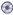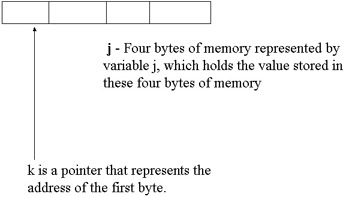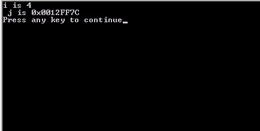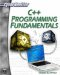# Chapter 9: PointersDownload CD Content

Pointers are a powerful, but troublesome part of C and C++. Most beginning C/C++ programmers have significant trouble understanding and appropriately using pointers, so please pay close attention to this chapter. The first, and most obvious question, is what, indeed, is a pointer? This question is probably foremost in your mind at this particular point in time. A pointer is a special type of variable, one that literally points to an address in memory of another variable. If you think about standard variables, they are labels used to identify small segments of memory set aside to hold data of a particular type. A pointer simply points to the segment of memory occupied by another variable. Whereas a standard variable holds a value, the value stored at an address in memory, a pointer simply holds the address of another variable. You probably noticed that this paragraph provided three different but similar definitions for the pointer. All three of these definitions say the same thing, but in slightly different ways. It is hoped that one of these definitions will strike a chord with you and you will comprehend the concept.

## Pointer Basics

As previously stated, a variable is a small section in memory set aside to hold data of a specific type. When you write:

`   int j; `

you have just set aside 4 bytes of memory and you are referring to that 4 bytes by the variable j. The variable j represents whatever value is currently stored in those 4 bytes of memory.

Declaring a pointer is almost the same. You still declare a data type, just as you do with standard variables; however, you must add the asterisk (*) symbol to denote that it is a pointer. You can place the asterisk immediately after the data type, or before the variable name. Either method is a valid way of declaring a pointer variable. For example, both of the following are valid pointer declarations.

`int* k; int *k;`

The asterisk(*) is called a reference operator. This name makes perfect sense, because it, indeed, is a reference to an address in memory. The * operator can be literally translated to “the address pointed at by,” The way you use a pointer is that you have it set equal to the address of another variable. Recall from Chapter 1 that the ampersand (&) operator denotes an address in memory, or is the “address of” operator. Let’s look at the following examples.

`int j; int *k: k = &j;`

Now the pointer k represents the address of j. More specifically it is pointing to the first byte of the 4 bytes that j occupies. This is illustrated in Figure 9.1.Figure 9.1: Pointers.

This is important to remember for several reasons. The first being that if you print-out j you will get a numeric value. However, if you print-out k you will get a rather long number that represents an address in memory. The following example illustrates this.

### Example 9.1

Step 1: Write the following code in your favorite text editor.

`#include <iostream> using namespace std;      int main()    {      int i;      int* j;      j = &i;      i = 4;      cout << "i is " << i;      cout << "\n j is " << j << "\n"; return 1;    }`

Step 2: Compile the code.

Step 3: Run the code. You should see something like what is shown in Figure 9.2Figure 9.2: Printing pointers.

You will note that when the pointer variable is printed out, a rather long number is displayed on the screen. This is NOT the value stored at a particular address in memory; rather, it is the actual numerical address of the first byte of the variable that the pointer points to. In addition to illustrating that pointers represent addresses, this should also help you understand why we use variables. Which is easier to remember, the variable j, or some lengthy address in memory?

 Hint! Pointers are typed. This means you must tell the compiler what type of variable your pointer is pointing to.

The fact that pointers are typed means that the following code would generate an error.

`char c = '0';   int *p = &c; `

This code will generate an error because you cannot use an int pointer to point to a variable of type char. Remember that you must use a pointer of the same data type as the variable it is pointing to.C++ Programming Fundamentals (Cyberrookies)
ISBN: 1584502371
EAN: 2147483647
Year: 2005
Pages: 197
Authors: Chuck Easttom